### Home > MC2 > Chapter 5 > Lesson 5.2.3 > Problem5-71

5-71.
1. Simplify each expression. Homework Help ✎

1.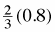2.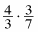3.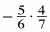4.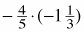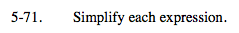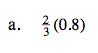Multiply and reduce.
Notice that 0.8 can be converted into a fraction.

$\left(\frac{2}{3}\right)\left(\frac{8}{10}\right)$

$\frac{8}{15}$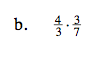Multiply and reduce.
Notice that since the two fractions are being multiplied the 3 in the numerator and the 3 in the denominator make a Giant One.

$\left(\frac{4}{1}\right)\left(\frac{1}{7}\right)$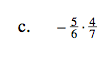Multiply and reduce.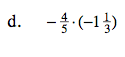Multiply and reduce.
Converting the second fraction into a fraction greater than one may prove to be more helpful.

$1\frac{1}{15}$